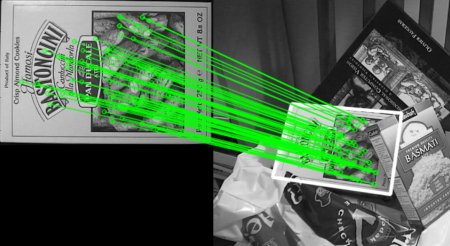OpenCV  3.1.0 Open Source Computer Vision
Feature Matching + Homography to find Objects

## Goal

In this chapter,

• We will mix up the feature matching and findHomography from calib3d module to find known objects in a complex image.

## Basics

So what we did in last session? We used a queryImage, found some feature points in it, we took another trainImage, found the features in that image too and we found the best matches among them. In short, we found locations of some parts of an object in another cluttered image. This information is sufficient to find the object exactly on the trainImage.

For that, we can use a function from calib3d module, ie cv2.findHomography(). If we pass the set of points from both the images, it will find the perpective transformation of that object. Then we can use cv2.perspectiveTransform() to find the object. It needs atleast four correct points to find the transformation.

We have seen that there can be some possible errors while matching which may affect the result. To solve this problem, algorithm uses RANSAC or LEAST_MEDIAN (which can be decided by the flags). So good matches which provide correct estimation are called inliers and remaining are called outliers. cv2.findHomography() returns a mask which specifies the inlier and outlier points.

So let's do it !!!

## Code

First, as usual, let's find SIFT features in images and apply the ratio test to find the best matches.

1 import numpy as np
2 import cv2
3 from matplotlib import pyplot as plt
4
5 MIN_MATCH_COUNT = 10
6
7 img1 = cv2.imread('box.png',0) # queryImage
8 img2 = cv2.imread('box_in_scene.png',0) # trainImage
9
10 # Initiate SIFT detector
11 sift = cv2.xfeatures2d.SIFT_create()
12
13 # find the keypoints and descriptors with SIFT
14 kp1, des1 = sift.detectAndCompute(img1,None)
15 kp2, des2 = sift.detectAndCompute(img2,None)
16
17 FLANN_INDEX_KDTREE = 0
18 index_params = dict(algorithm = FLANN_INDEX_KDTREE, trees = 5)
19 search_params = dict(checks = 50)
20
21 flann = cv2.FlannBasedMatcher(index_params, search_params)
22
23 matches = flann.knnMatch(des1,des2,k=2)
24
25 # store all the good matches as per Lowe's ratio test.
26 good = []
27 for m,n in matches:
28  if m.distance < 0.7*n.distance:
29  good.append(m)

Now we set a condition that atleast 10 matches (defined by MIN_MATCH_COUNT) are to be there to find the object. Otherwise simply show a message saying not enough matches are present.

If enough matches are found, we extract the locations of matched keypoints in both the images. They are passed to find the perpective transformation. Once we get this 3x3 transformation matrix, we use it to transform the corners of queryImage to corresponding points in trainImage. Then we draw it.

1 if len(good)>MIN_MATCH_COUNT:
2  src_pts = np.float32([ kp1[m.queryIdx].pt for m in good ]).reshape(-1,1,2)
3  dst_pts = np.float32([ kp2[m.trainIdx].pt for m in good ]).reshape(-1,1,2)
4
5  M, mask = cv2.findHomography(src_pts, dst_pts, cv2.RANSAC,5.0)
7
8  h,w,d = img1.shape
9  pts = np.float32([ [0,0],[0,h-1],[w-1,h-1],[w-1,0] ]).reshape(-1,1,2)
10  dst = cv2.perspectiveTransform(pts,M)
11
12  img2 = cv2.polylines(img2,[np.int32(dst)],True,255,3, cv2.LINE_AA)
13
14 else:
15  print "Not enough matches are found - %d/%d" % (len(good),MIN_MATCH_COUNT)

Finally we draw our inliers (if successfully found the object) or matching keypoints (if failed).

1 draw_params = dict(matchColor = (0,255,0), # draw matches in green color
2  singlePointColor = None,
4  flags = 2)
5
6 img3 = cv2.drawMatches(img1,kp1,img2,kp2,good,None,**draw_params)
7
8 plt.imshow(img3, 'gray'),plt.show()

See the result below. Object is marked in white color in cluttered image:image